# Quadratic Equations Shortcuts with Examples

An equation in which the highest power of the variable is 2 is called a quadratic equation.For example, the equation of the type ax2+bx+c=0 denotes a quadratic equation.
There are many ways for solving a quadratic equations  but while in exams we need a quick answer
so there is a shortcut method which used for solving a quadratic equations in less time but before let me tell you the most basic and accurate method which is used for it.

When we solved a quadratic expression or equation, it will always gives two values of variable. these values are called roots of the equation or expression.
1. By taking square roots or Factor Method

#### 1. Factorization of Quadratic EquationCondition for Factorization of a quadratic Equation
1. If b2 - 4ac  > 0, then the quadratic equation can be factorized.
2. If b2 - 4ac  < 0, then the quadratic equation cannot be factorized.
some basic formulas which used in while solving through factorization method.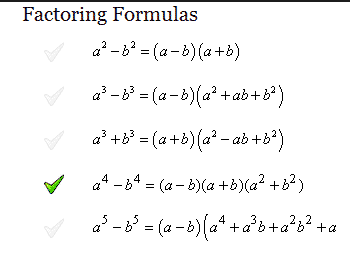Discriminant of ax2+bx+c = 0 is D = b2 - 4ac and the two values of x obtained from a quadratic equation are called roots of the equation which denoted by α and β sign.
For a quadratic equations ax2+bx+c = 0
If α,β are the roots of ax2 + bx + c = 0 then
α = ( - b + √ b2 - 4ac ) /2a
β = ( - b -√ b2 - 4ac ) /2a .
1. When D = 0 ,Both the roots will be real an equal ( a = b ) and rational.
2. When D > 0 but not perfect square then the roots will be irrational, unequal and real .
3. When D > 0 and perfect square then the roots will be rational, unequal and real .
4. When D < 0 imaginary roots
Example:
x2 - 4x + 3 = 0  ; a = 1, b= -4, c = 3
D = b- 4ac = (-4)2 -4×1×3 = 16-12 = 4 > 0 and also a perfect square. So, the roots will be distinct rational numbers.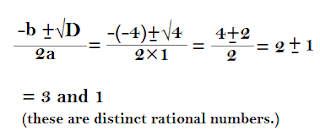when you have the roots and you need to make a quadratic equation from that roots then formula that is used  = x- x (sum of the roots)+product of the roots = 0
Now let you have roots 2 and 3 then the quadratic equation will be using formula( x2-x(sum of the roots)+product of the roots = 0 ) is : x2 - (2+3)×x + (3×2) = 0 ; x2-5x+6 = 0 .
Note:
• A quadratic equation ax2 + bx + c = 0 will have reciprocal roots, if a =c .
• When a quadratic equation ax2 + bx + c = 0 has one root equal to zero, then c = 0.
• When both the roots are equal to zero, b = 0 and c =0.
• When the roots of the quadratic equation ax2+bx = c are negative reciprocals of each other, then c = -a .
• If they have both the roots common, then a/a1 + b/b1 + c/c1.
• The square root of any negative number will be an imaginary number like √-25 = √(-1)× 25 = √-1×√ 25 = 5i
Example :
Forming a quadratic equation whose roots are 3 and 5 and verifying them.
the equation will be
x2 - sum of the roots × x + products of the roots = 0
x2 - { 3+ (-5)}×x + 3×(-5) = 0
x2 - (-2)x+(-15) = 0 or x2 + 2x - 15 = 0
Verification : D = 4+ 60 = 64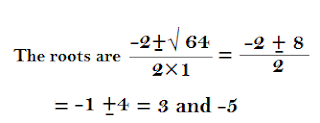### Solving Quadratic Equation using shortcut techniques

#### Direction (1-3) : In each question, one or more equations are provided. On the basis of these, you have to find out relation between p and q.

Give answer (a) if p = q , Give answer (b) if p > q , Give answer (c) if q > p , Give answer (d) if p ≥ q and give answer (e) if q ≥ p .
Q1. (i) 4p2 - 5p +1 = 0 (ii) q2-2q+1 = 0
Sol :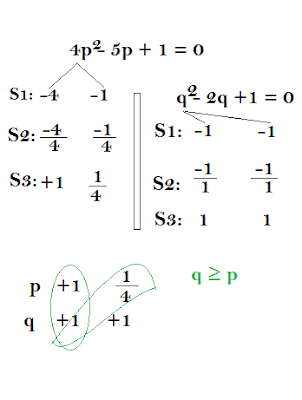Q2. (i) q2 - 11q + 30 = 0 (ii) 2p2 - 7p + 6=0
Sol:Q3. (i) 6q2 + 1/2 = 7/2 q (ii) 12p2 + 2 = 10p
Sol:

(i) 6q2 + 1/2 = 7/2 q => 12q- 7q + 1 = 0
(ii) 12p2 + 2 = 10p => 6p2 - 5p + 1 = 0

Q4. x - 1/x = y, then x+ 1 / x= ?
Sol:Q5. If (x-1) and (x+1) be the sides (in cm) of a right triangle, then the value of x is ?
Sol:
(H)2 =  (P)2 + B)2
(x+1)2 = (x)2 + (x-1)2
x2+1+2x = x2 + x +1 - 2x
-x2 + 4x = 0
x = 4
Q6. Two consecutive positive even integers whose squares have the sum 164 are ?
Sol:
(x)2 + (x+2)2 = 164
x2+x2+4+4x = 164
2x2+4x+4-164 = 0
2(x2+2x-80) = 0
x2+2x-80 = 0
(x2+10-8x-80 = 0
(x-8) (x+10) = 0
x = 8 , x = -10
hence numbers 8 & 10.
Q7. The sum of two numbers is 15 and the sum of their reciprocals is 3/10 then the numbers are ?
Sol:
x+y = 15
1/x + 1/y = 3/10
x = 15 - y
1/15-y + 1/y = 3/10
150 = 45y - 3y2
3y2 - 45y + 150 = 0
3(y2 - 15y +50 ) = 0
(y-10) (y-5) = y = 10 , y = 5.
Q8. If the length of a rectangle is square of the breadth and area is 64 find the length ?
Sol:
Let Breadth  = x, Length = x2
Area = x3
x3 = 64, x = 4 , B = 4, L = 16
Q9. If x+ 1 / x= 102 , then the value of x - 1/x =?
Sol :Q10. The sum of a number and its reciprocal is 17/4, then the number is ?
Sol:Q11. The hypotenus of a right angled triangle is 1 m less than 2 times the smallest side. If the third side is 1 m more than the smallest then sides of the triangle are ?
Sol :
B = x
H = 2x -1
P = x + 1
( 2x - 1 )2 = x2 + ( x+ 1 )2
4x+ 1 - 4x = x2 + x+ 1 + 2x
4x+ 1 - 4x = 2x+ 2x +1
2x2 - 6x = 0
x = 3,
H = 2(3) - 1 = 5
P = 3 + 1 = 4
Q12. The solution of √25-x= x-1 are
Sol: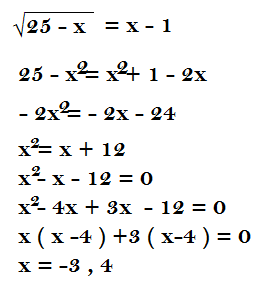Q13. If x - ax + 20 is exactly divisible by x-4 then a = ?
Sol :
x2- ax + 20
x- 4 ; x =4
(4)2 - a (4) + 20 = 0
16 - 4a + 20 = 0
-4a + 36 = 0
a = 36/ 4 = 9.#### What's trending in BankExamsToday

Smart Prep Kit for Banking Exams by Ramandeep Singh - Download here